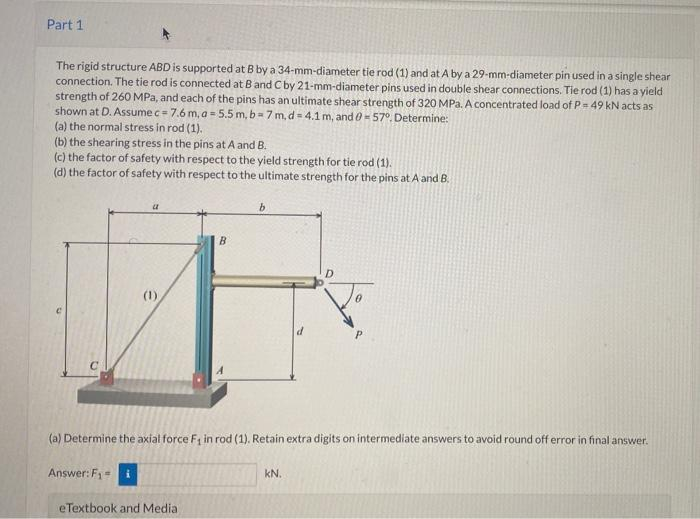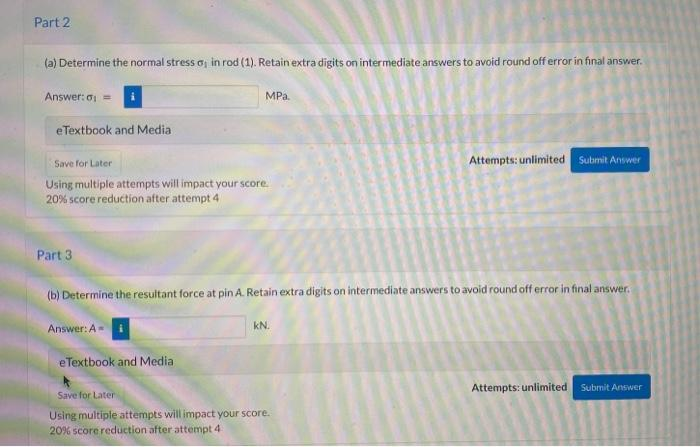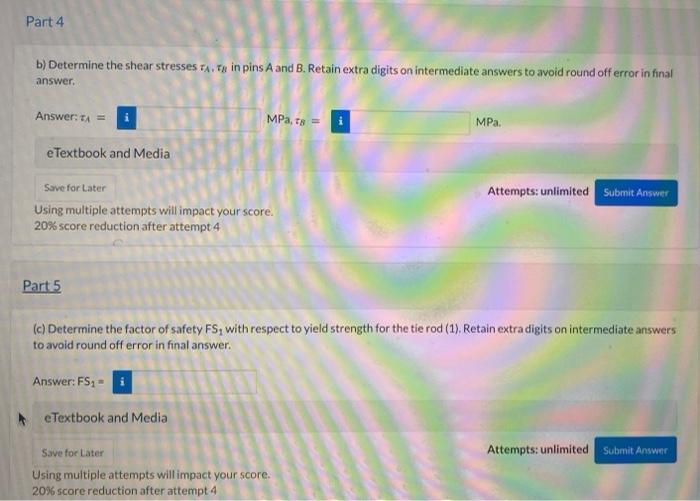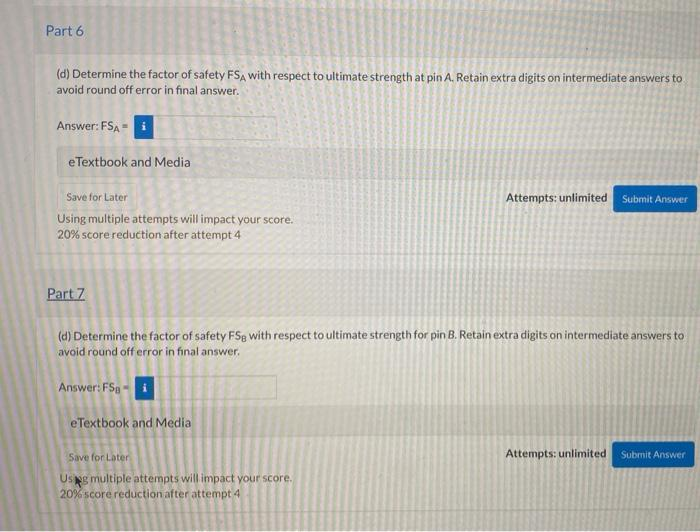Home / Expert Answers / Mechanical Engineering / the-rigid-structure-abd-is-supported-at-b-by-a-34-mm-diameter-tie-rod-1-and-at-a-by-a-29-pa555

# (Solved): The rigid structure ABD is supported at B by a 34-mm-diameter tie rod (1) and at $$A$$ by a $$29 ...The rigid structure ABD is supported at B by a 34-mm-diameter tie rod (1) and at \( A$$ by a $$29-\mathrm{mm}$$-diameter pin used in a single shear connection. The tie rod is connected at B and C by 21-mm-diameter pins used in double shear connections. Tie rod (1) has a yield strength of $$260 \mathrm{MPa}$$, and each of the pins has an ultimate shear strength of $$320 \mathrm{MPa}$$. A concentrated load of $$P=49 \mathrm{kN}$$ acts as shown at D. Assume $$c=7.6 \mathrm{~m}, a=5.5 \mathrm{~m}, b=7 \mathrm{~m}, d=4.1 \mathrm{~m}$$, and $$\theta=57^{\circ}$$. Determine: (a) the normal stress in rod (1). (b) the shearing stress in the pins at $$A$$ and $$B$$. (c) the factor of safety with respect to the yield strength for tie rod (1). (d) the factor of safety with respect to the ultimate strength for the pins at $$A$$ and $$B$$, (a) Determine the axial force $$F_{1}$$ in rod (1). Retain extra digits on intermediate answers to avoid round off error in final answer. Answer: $$F_{1}=$$ $$\mathrm{kN}$$. (a) Determine the normal stress $$\sigma_{1}$$ in rod (1). Retain extra digits on intermediate answers to avoid round off error in final answer. Answer: $$\sigma_{1}=$$ MPa. eTextbook and Media Attempts: unlimited Using multiple attempts will impact vour score. $$20 \%$$ score reduction after attempt 4 Part 3 (b) Determine the resultant force at pin A. Retain extra digits on intermediate answers to avoid round off error in final answer. Answer: $$\mathrm{A}=$$ b) Determine the shear stresses $$\tau_{A}, \tau_{B}$$ in pins $$A$$ and B. Retain extra digits on intermediate answers to avoid round off error in final answer. Answer: $$\tau_{A}=$$ eTextbook and Media $$\mathrm{MPa}_{,} \mathrm{\tau}_{B}=$$ $$\mathrm{MPa}$$. Attempts: unlimited Using multiple attempts will impact your score. $$20 \%$$ score reductionafter attempt 4 Parts (c) Determine the factor of safety $$\mathrm{FS}_{1}$$ with respect to yield strength for the tie rod (1). Retain extra digits on intermediate answers to avoid round off error in final answer. Answer: $$\mathrm{FS}_{1}=$$ (d) Determine the factor of safety FS A with respect to ultimate strength at pin $$A$$. Retain extra digits on intermediate answers to avoid round off error in final answer. Answer: $$\mathrm{FS}_{\mathrm{A}}=$$ eTextbook and Media Save for Later Attempts: unlimited Using multiple attempts will impact your score. $$20 \%$$ score reduction after attempt 4 Part7 (d) Determine the factor of safety $$\mathrm{FS}_{\mathrm{B}}$$ with respect to ultimate strength for pin $$\mathrm{B}$$. Retain extra digits on intermediate answers to avoid round off error in final answer. Answer: $$F_{S}=$$ eTextbook and Media

We have an Answer from Expert